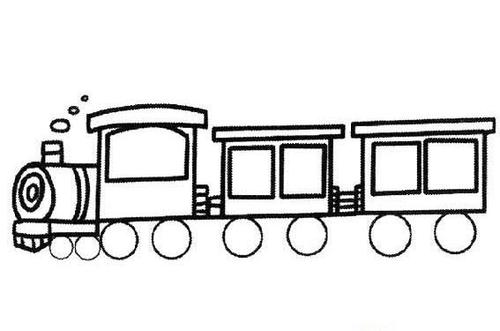## # 1. 什么是链表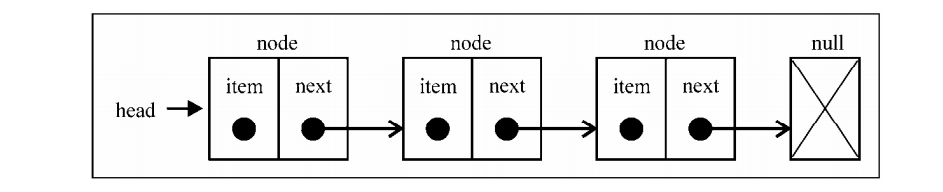### # 1.1 链表的优点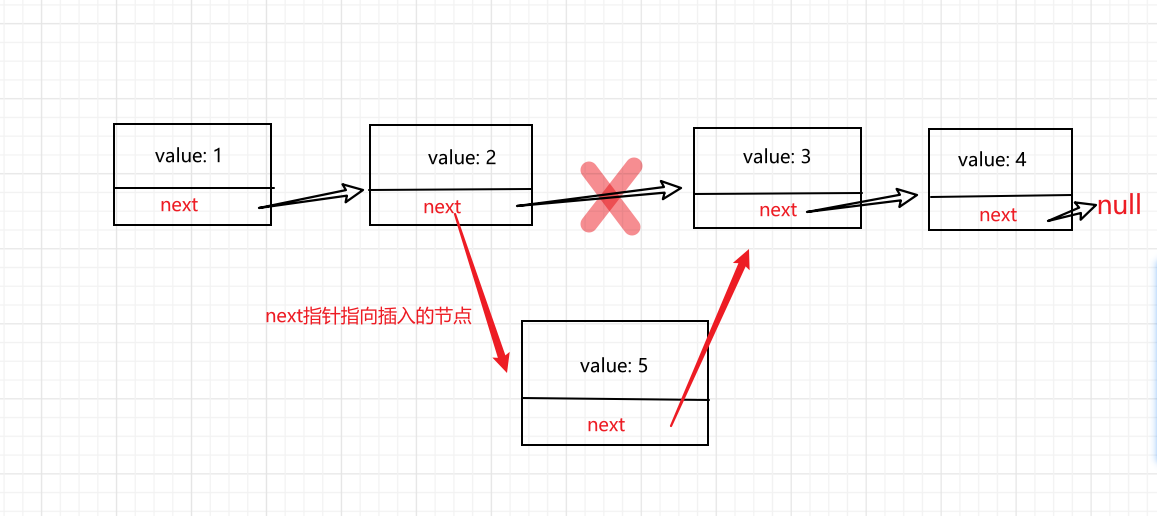### # 1.3 单向链表与数组各个操作时间复杂度对比

search（访问） `O(n)` search（访问） `O(1)`
insert（插入） `O(1)` insert（插入） `O(n)`
remove（删除） `O(1)` remove（删除） `O(n)`
append （添加） `O(1)` append （添加） `O(1)`

### # 1.4 链表的分类

#### # 1.4.1 单向链表#### # 1.4.2 双向链表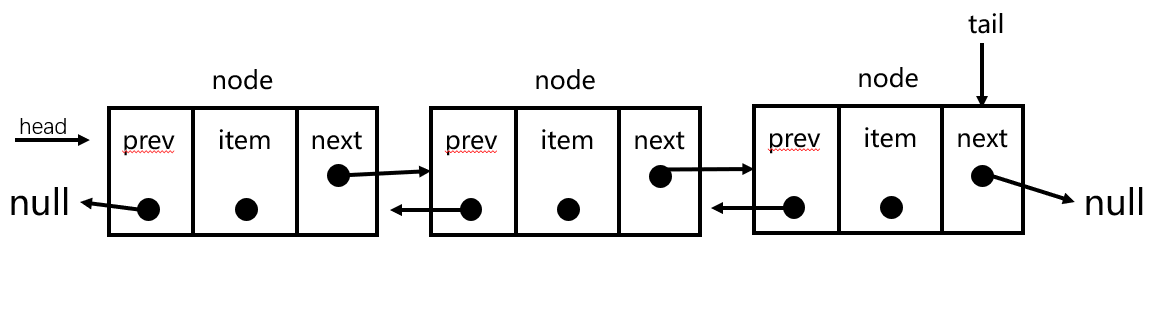#### # 1.4.3 循环链表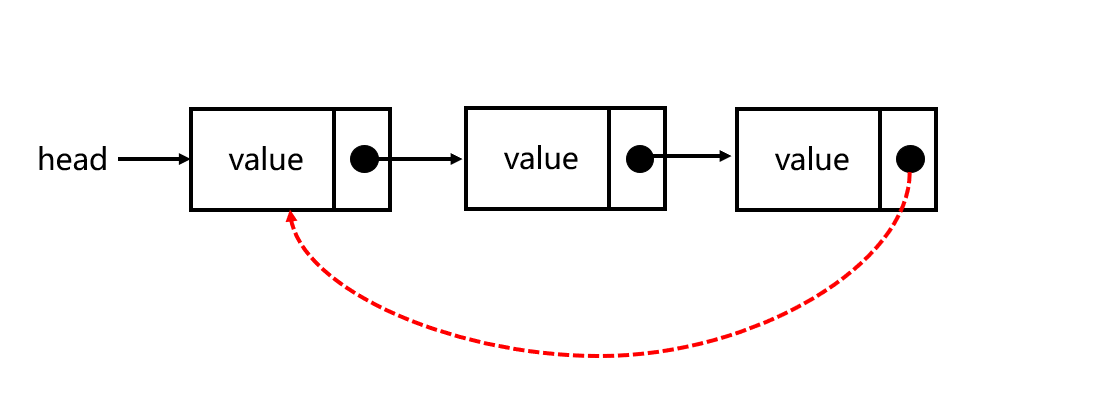## # 2. 使用JS实现链表

### # 2.1 单向链表

#### # 2.1.1 创建一个单向链表

``````
constructor() {
// 链表的长度size
this.size = 0;
}
//添加链表项
append(element) { }
//插入链表项
appendAt(position, element) { }
// 删除指定列表项
remove(element) { }
//删除指定位置链表项
removeAt(position) { }
// 查找指定元素索引
indexOf(element) { }
// 翻转链表
reserve() { }
// 获取节点
getNode(index) {}
}
//测试
``````
1
2
3
4
5
6
7
8
9
10
11
12
13
14
15
16
17
18
19
20
21
22
23
24

``````// node类
class Node {
constructor(element) {
this.element = element;
// 添加一个指针
this.next = null
}
}
``````
1
2
3
4
5
6
7
8

• `append(element)` ：向链表尾部添加一个新的项
• `appendAt(position, element)` ： 向链表的特定位置插入一个新的项
• `remove(element)`：从列表中移除一项
• `removeAt(position)`：从列表的特定位置移除一项
• `getNode(index)`：获取某个位置的节点
• `reserve()`：反转链表

#### # 2.1.2 获取链表中的节点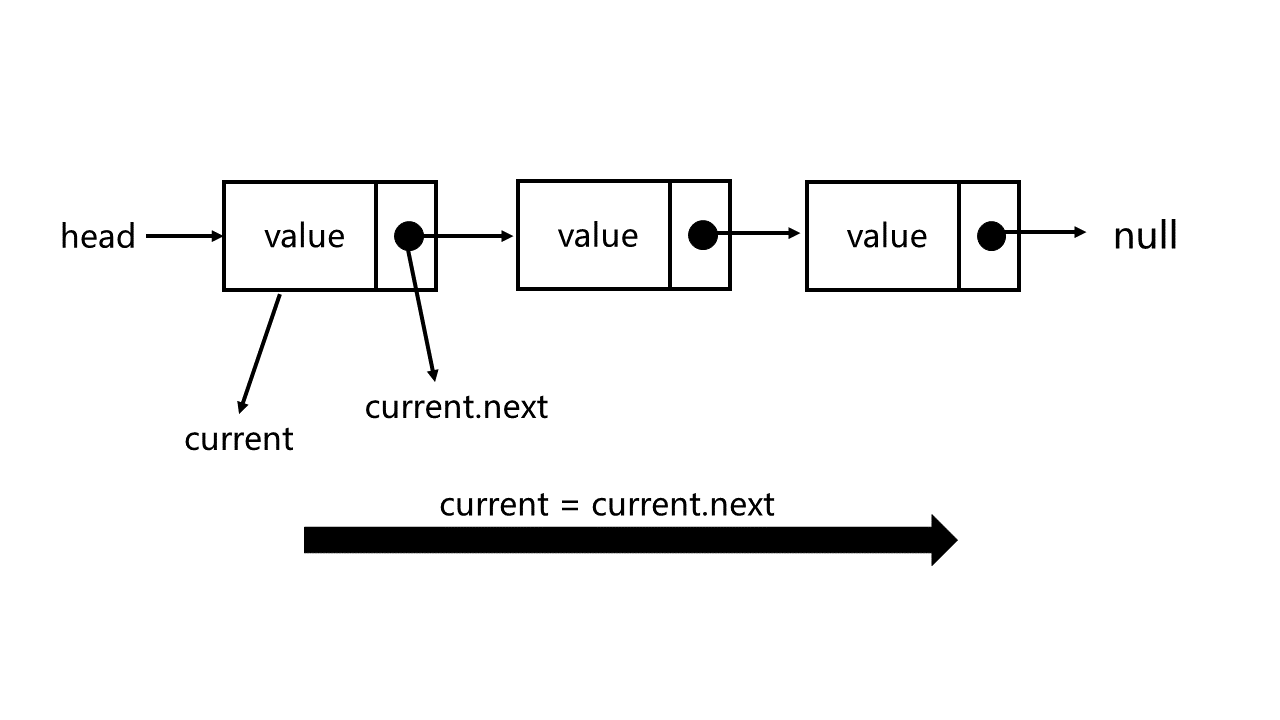``````getNode(index) {
// 边界判断 传入的参数是否在链表的长度范围之内
if (index < 0 || index >= this.size) {
throw new Error('out')
}
// 不断的让current移到下一位，直到到达index - 1位置
// 也就是循环遍历的过程
for (let i = 0; i < index; i++) {
current = current.next;
}
// 最后返回节点
return current
}
``````
1
2
3
4
5
6
7
8
9
10
11
12
13
14

#### # 2.1.3 向链表尾部追加元素

1. 列表为空，添加的是第一个元素
2. 列表不为空，向其追加元素

``````append(element) {
// 通过实例化添加属性
let node = new Node(element);
// 添加操作
} else {
let current = this.getNode(this.size - 1);
// 最后一个节点的next指向新创建的节点
current.next = node
}
//添加链表项后，size自增
this.size++;
}
``````
1
2
3
4
5
6
7
8
9
10
11
12
13
14

#### # 2.1.4 向链表的任意位置插入一个元素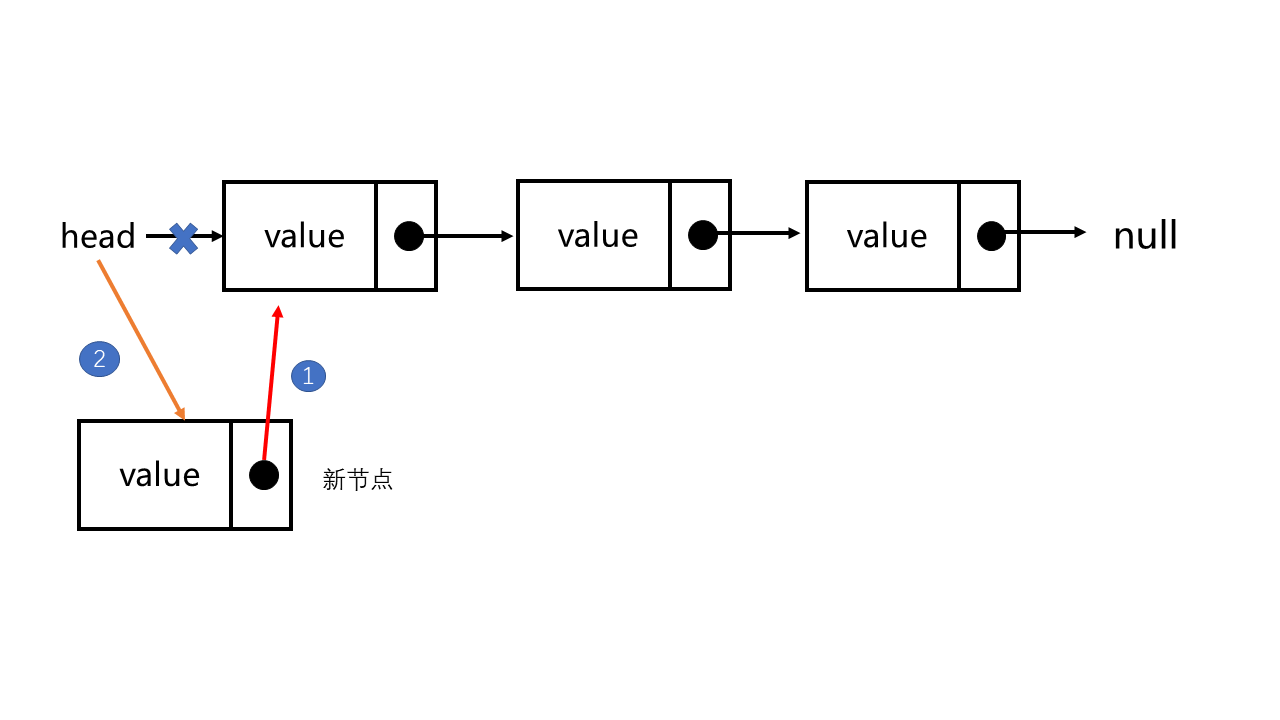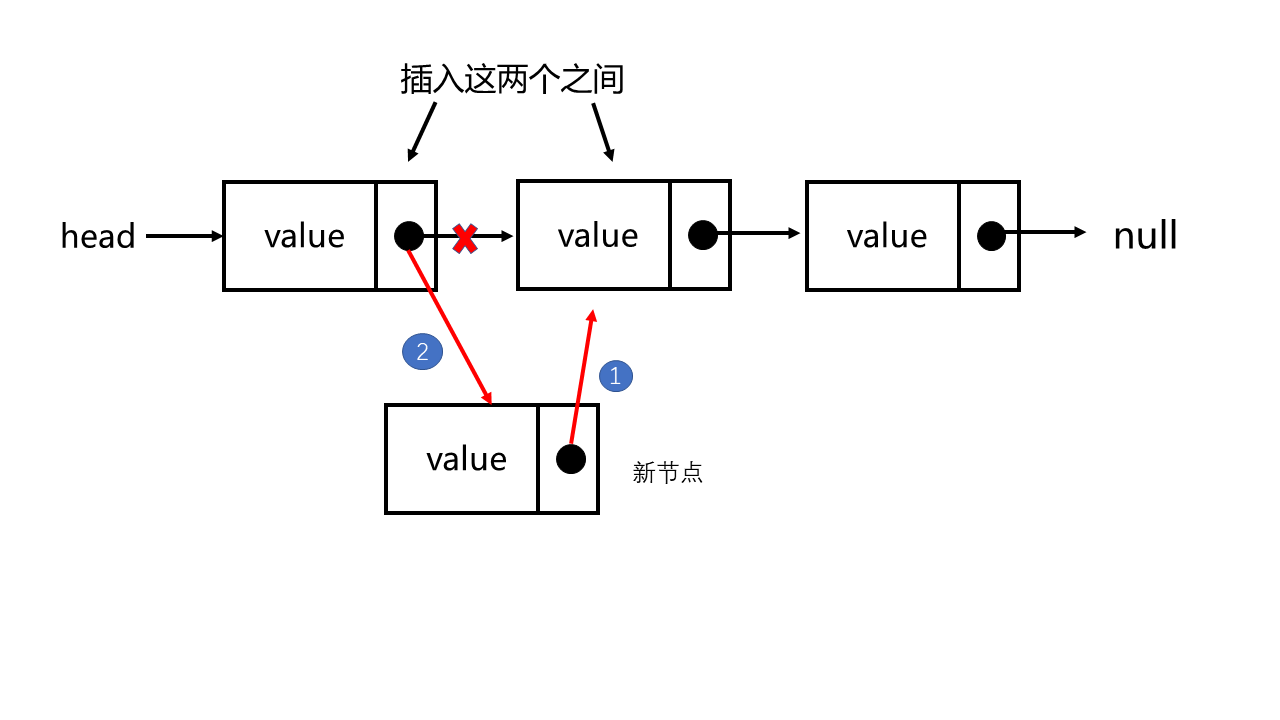``````//插入链表项
appendAt(position, element) {
// 边界判断
if (position < 0 || position > this.size) {
throw new Error('position out range')
}
// 实例化创建节点
let node = new Node(element);
//起点插入
if (position === 0) {
} else {
// 找到需要插入位置的前一个节点
let pre = this.getNode(position - 1);
// 让新创建的节点指向当前前一个节点的下一个节点
node.next = pre.next;
// 让前一个节点指向新创建的节点
pre.next = node;
}
}
``````
1
2
3
4
5
6
7
8
9
10
11
12
13
14
15
16
17
18
19
20
21

#### # 2.1.5 从链表中移除元素（根据特定位置移除）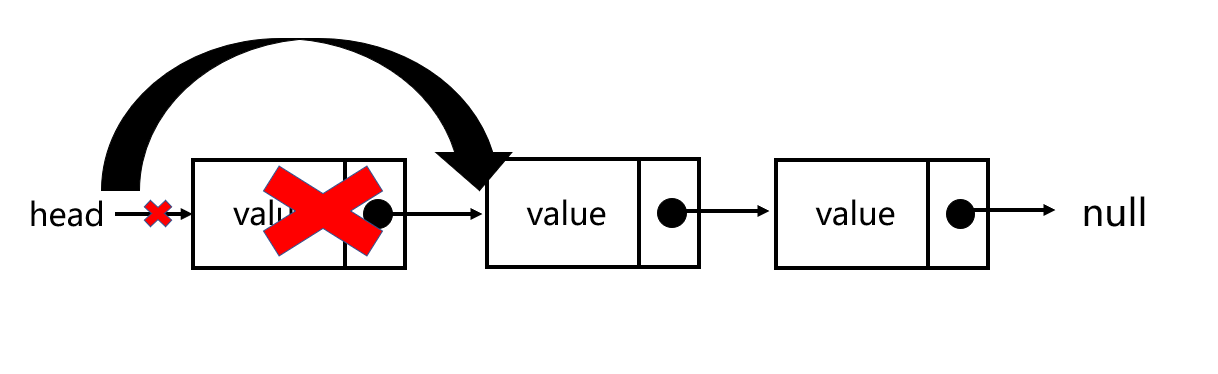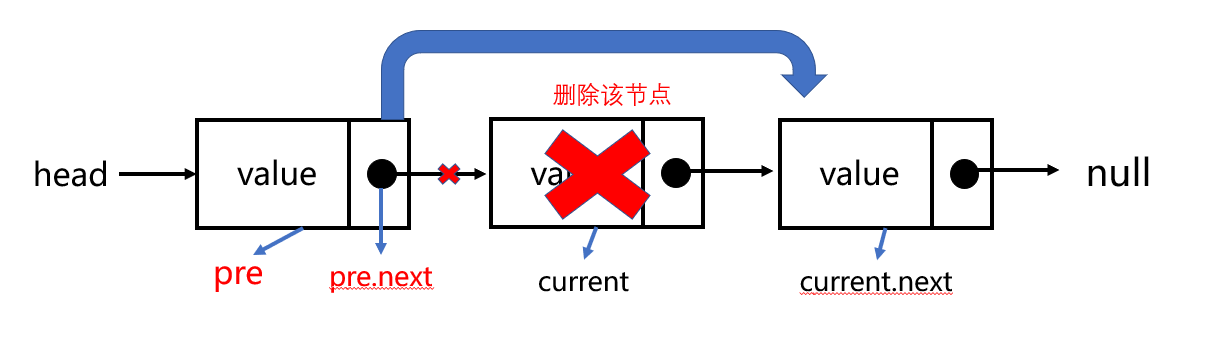``````//删除指定位置链表项
removeAt(position) {
// 边界判断，超出链表长度，抛出错误
if (position < 0 || position >= this.size) {
throw new Error('position out range')
}
if (position === 0) {
} else {
// 获取删除位置的前一个节点
let pre = this.getNode(position - 1);
// current为被删除节点
current = pre.next;
// 让前一个节点指向删除节点的下一个节点
pre.next = current.next;
}
// 长度自减
this.size--;
}
``````
1
2
3
4
5
6
7
8
9
10
11
12
13
14
15
16
17
18
19
20
21

#### # 2.1.6 查找元素在链表的位置

``````// 查找指定元素索引
indexOf(element) {
// 当前链表头节点
// 遍历整个链表
for (let i = 0; i < this.size; i++) {
if (current.element === element) {
return i
}
// 依次向下移动
current = current.next;
}
return -1;
}
``````
1
2
3
4
5
6
7
8
9
10
11
12
13
14

#### # 2.1.7 根据元素值移除元素

``````remove(element) {
// 获取需要删除元素在链表的位置
let index = this.indexOf(element)
if(index!= -1 ) {
// 通过下面的removeAt方法删除指定位置节点
this.removeAt(index)
}else {
// 删除不存在的元素，抛出错误
throw new Error('element no found')
}
}
``````
1
2
3
4
5
6
7
8
9
10
11

#### # 2.1.8 反转链表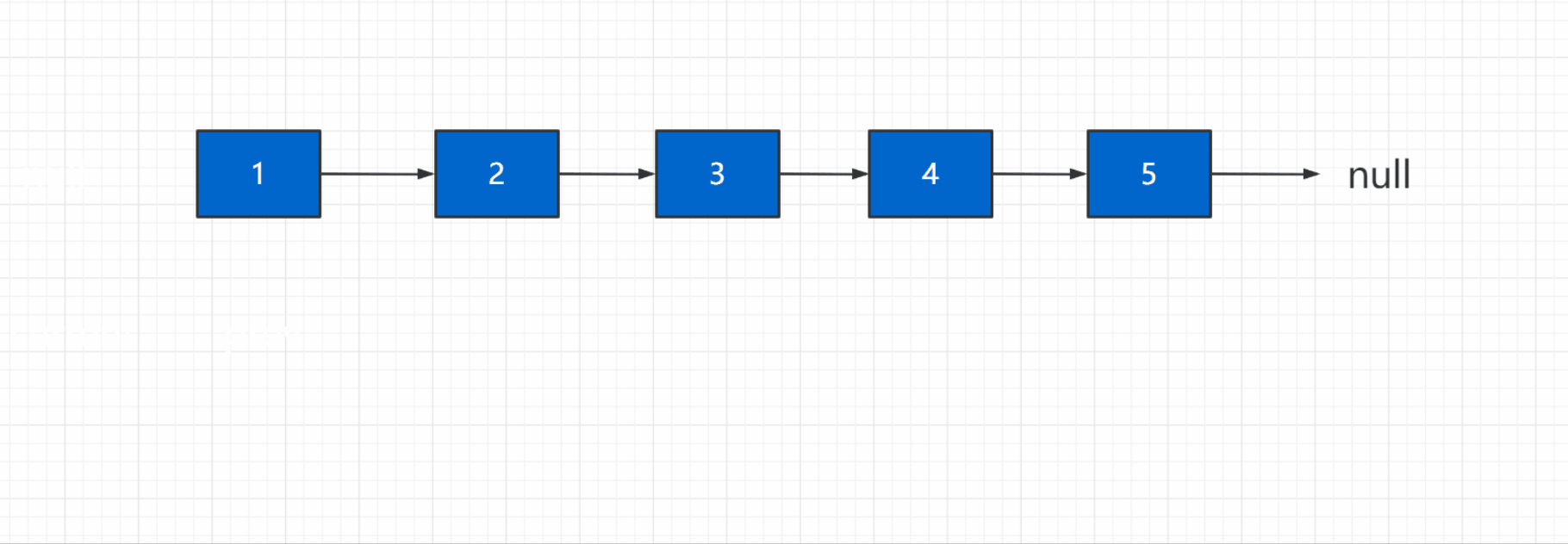``````reserve() {
let prev = null;
while (current) {
// 保存当前的下一位
let next = current.next;
// 让当前节点指向前面的节点prev
current.next = prev;
// 交换完之后，让prev移到下一位也就是current
prev = current;
current = next;
}
//返回翻转的链表
}
``````
1
2
3
4
5
6
7
8
9
10
11
12
13
14
15

### # 2.2 双向链表#### # 2.2.1 创建一个双向链表

``````//一个链表节点
class Node {
constructor(element) {
this.element = element
this.next = null
this.prev = null//新增
}
}
//双向链表
constructor () {
this.size = 0
this.tail = null//新增
}
// 这里是接下来写的方法
}
``````
1
2
3
4
5
6
7
8
9
10
11
12
13
14
15
16
17

#### # 2.2.2 获取链表中的节点

``````// 获取某个位置的节点，和单向链表相同
getNode(index) {
if (index < 0 || index >= this.size) {
throw new Error('out')
}
for(let i = 0;i < index;i++) {
current = current.next
}
return current
}
``````
1
2
3
4
5
6
7
8
9
10
11

#### # 2.2.3 向链表尾部追加节点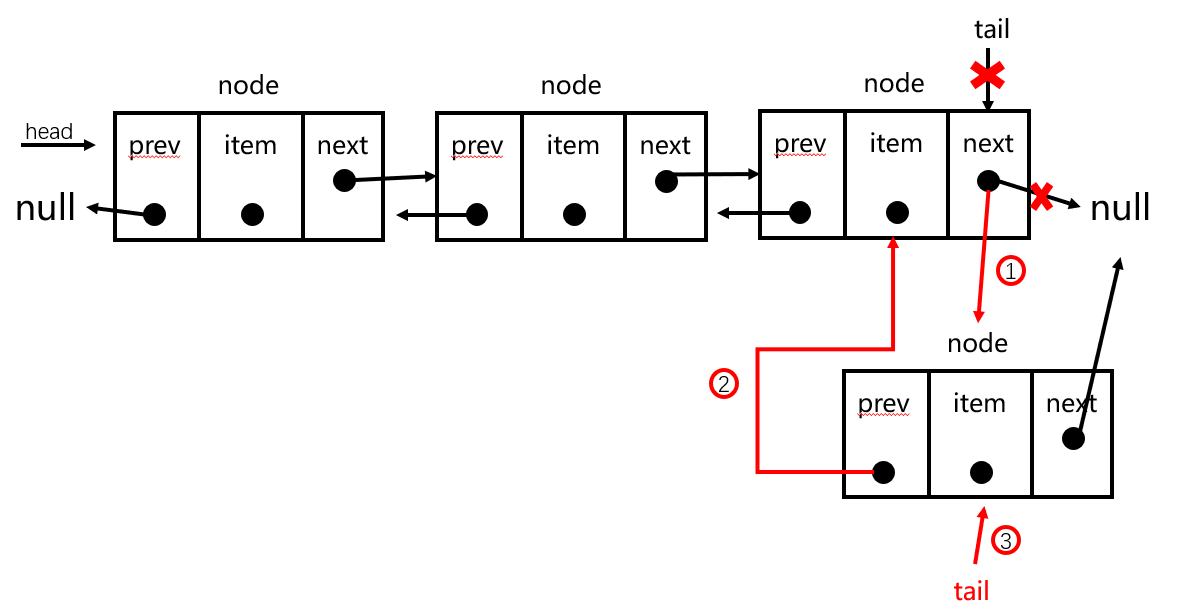``````append(element) {
// 创建节点
let node = new Node(element)
// 保存当前指向最后一个元素的指针
let tail = this.tail
// 如果链表为空则新节点将作为第一个
this.tail = node
}else {
// 常规尾插，让最后一个元素的next指针指向node
tail.next = node
// 再让node的前驱指针指向最后一个节点tail
node.prev = tail
// 最后更新tail指向最后一个元素node
this.tail = node
}
this.size++
}
``````
1
2
3
4
5
6
7
8
9
10
11
12
13
14
15
16
17
18
19
20

#### # 2.2.4 向链表中插入节点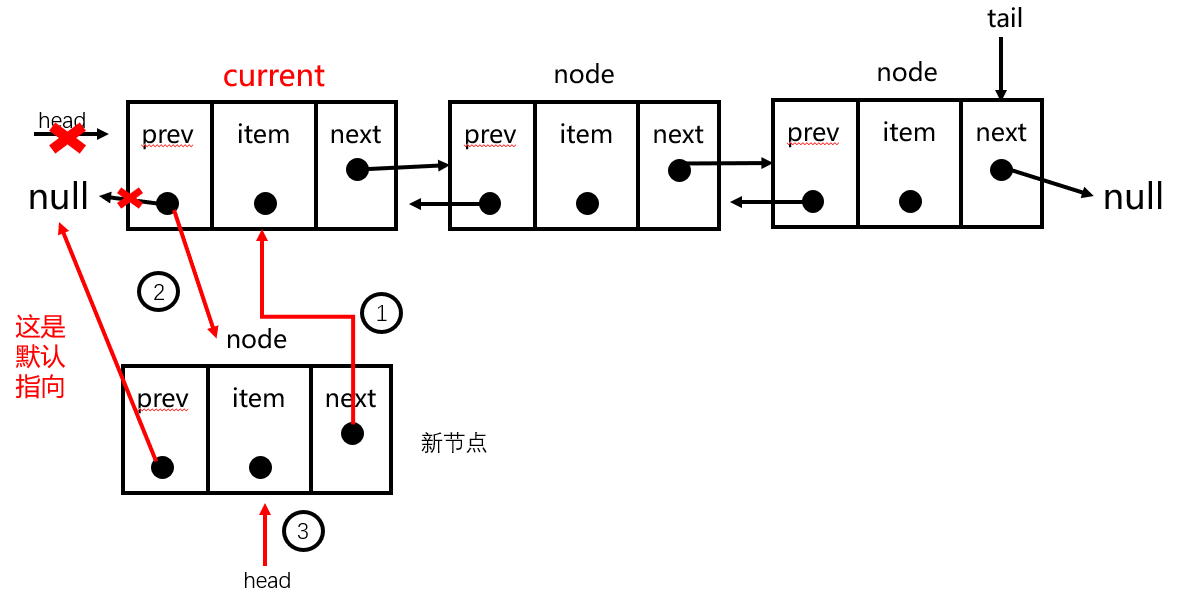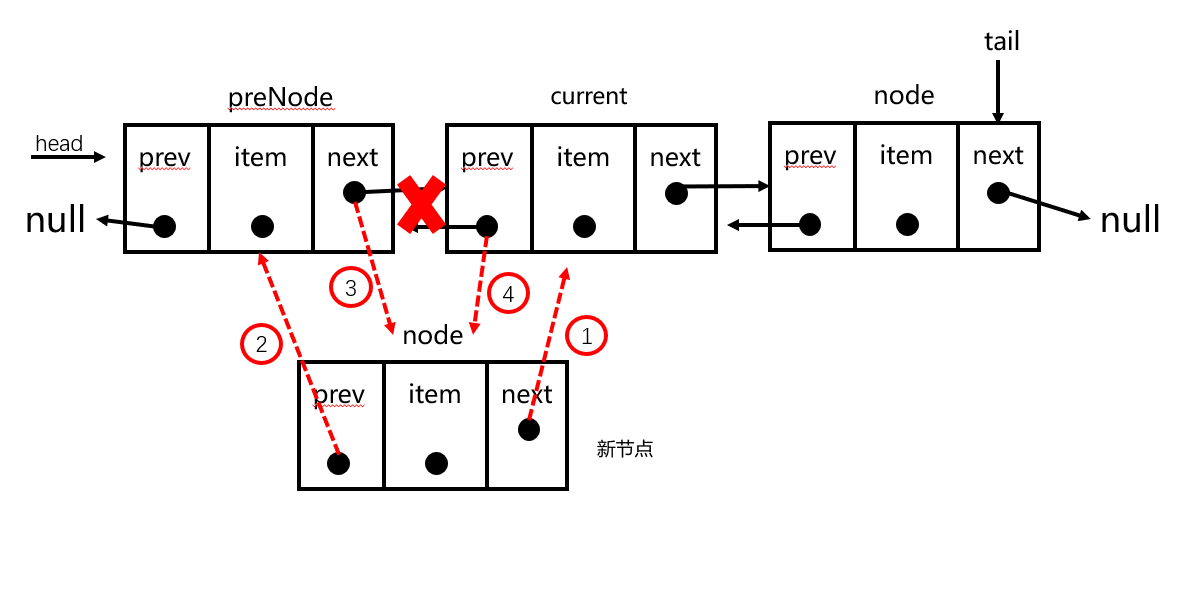``````// 插入节点
insert(position, element) {
// 边界判断，输入的position需要在范围之内
if (position < 0 || position > this.size) {
throw new Error('position out range')
}
// 创建节点
let node = new Node(element)
// 保存第一个节点，为一个变量
// 第一种情况，在最头部插入
if (position === 0) {
// 链表为空的情况
this.tail = node
} else {
// 链表不为空
// 让node的next指针指向第一个节点
node.next = current
// 第一个节点的前驱指针指向node
current.prev = node
}
this.size++
} else if (position === this.size) {
// 在最后插入
// current变量保存最后一个节点
current = this.tail
// 最后一个节点的next指针指向新节点
current.next = node
// 新节点的前驱指针指向前一个节点
node.prev = current
// tail移至node
this.tail = node
this.size++
} else {
// 在中间插入
// 获取插入位置的前一个节点
let preNode = this.getNode(position - 1)
// current变量保存preNode节点的下一个节点
current = preNode.next
// 1. 让node节点的next指针指向后一个节点current
node.next = current
// 2. 让node节点的prev指针指向前一个节点preNode
node.prev = preNode
// 3. 让preNode节点的next指针指向新节点
preNode.next = node
// 4. 让current节点的prev指针指向新节点
current.prev = node
this.size++
}
}
``````
1
2
3
4
5
6
7
8
9
10
11
12
13
14
15
16
17
18
19
20
21
22
23
24
25
26
27
28
29
30
31
32
33
34
35
36
37
38
39
40
41
42
43
44
45
46
47
48
49
50
51
52
53
54

#### # 2.2.5 从链表中的特定位置删除元素

`current`变量保存链表中第一个节点的引用，也就是我们想要移除的第一个节点。首先要改变的是 `head` 的引用，将其从`current`改为`current.next`。但我们还需要更新`current.next`指向上一个元素的指针，因此需要判断链表的长度是否为1，如果为1说明删除第一个节点之后链表就为空了，这时候就需要将`tail`设为`null`，如果不为1，则把`head.prev`的引用改为`null`即可。演示图如下：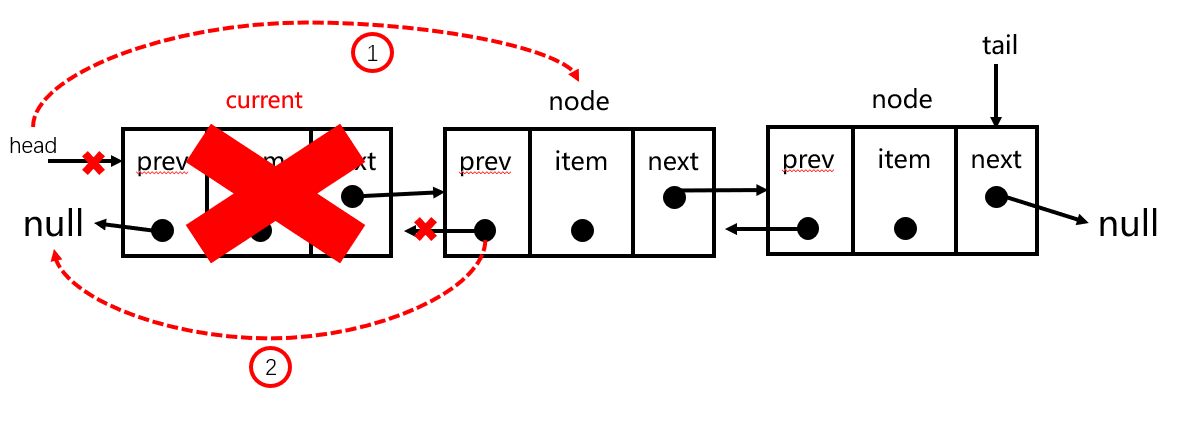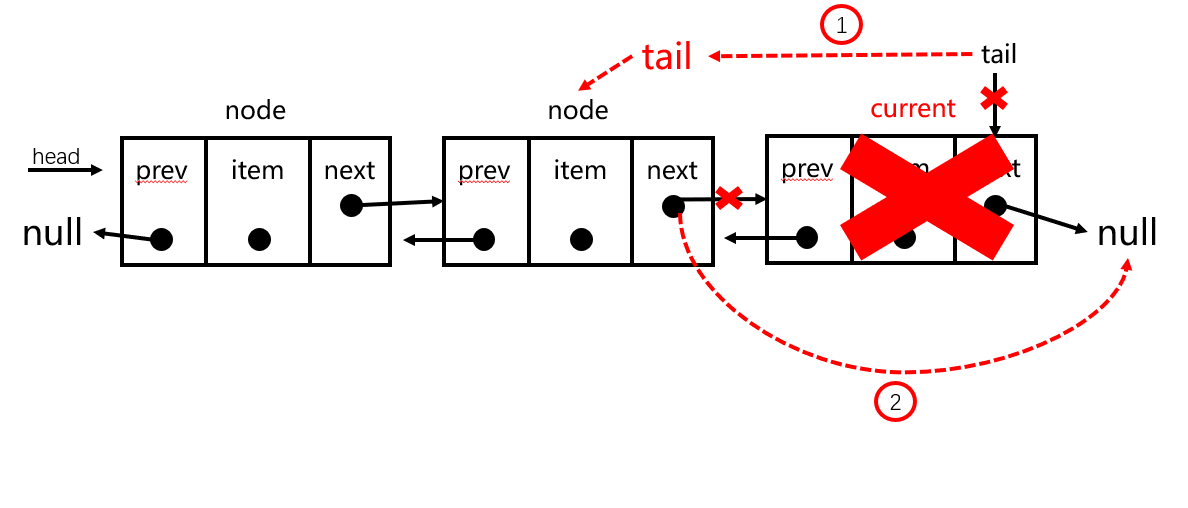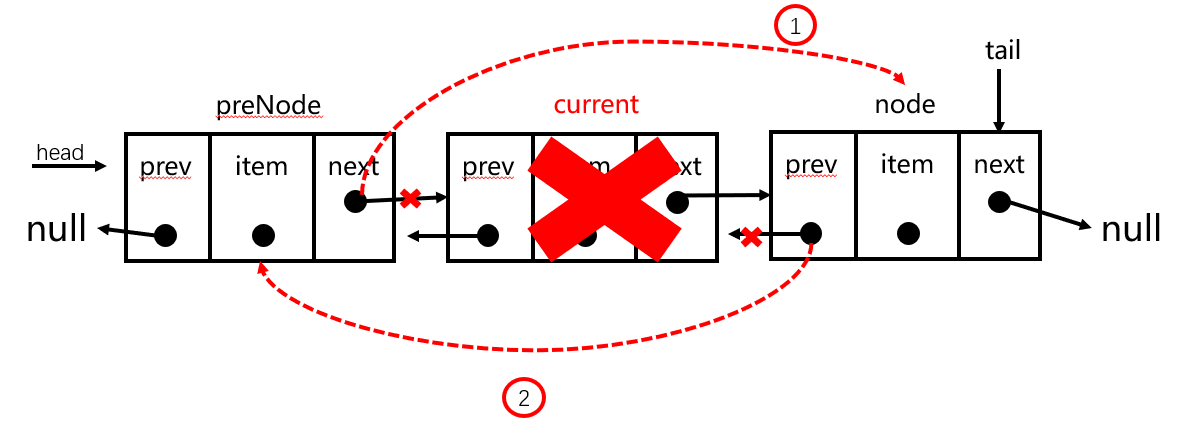``````removeAt(position) {
// 边界判断，输入的position需要在范围之内
if (position < 0 || position > this.size) {
throw new Error('position out range')
}
// 如果删除的是第一个节点
if (position === 0) {
// 如果链表的长度为1，删除后末尾应当为null
if(this.size === 1) {
this.tail = null
}else {
// 不为1 删除节点后第一个节点（current.next)指向null
}
this.size--
}else if (position === this.size -1) {
// 删除最后一个节点
// 先让current保存我们要删除的节点
current = this.tail
// 让tail移向删除节点的前一位
this.tail = current.prev
// 再让tail的next指针指向null，这样最后一个节点就被丢弃了
this.tail.next = null
this.size--
}else {
// 被删除的节点
let current = this.getNode(position)
// 删除节点的前一个节点
let preNode = current.prev
// 删除节点的前一个节点next指向它的后一个节点
preNode.next = current.next
// 后一个节点的prev指向preNode
current.next.prev = preNode
this.size--
}
}
``````
1
2
3
4
5
6
7
8
9
10
11
12
13
14
15
16
17
18
19
20
21
22
23
24
25
26
27
28
29
30
31
32
33
34
35
36
37
38
39

#### # 2.2.6 查找元素在链表中的位置

``````indexOf(element) {
// 当前链表头节点
// 遍历整个链表
for (let i = 0; i < this.size; i++) {
if (current.element === element) {
return i
}
// 依次向下移动
current = current.next;
}
return -1;
}
``````
1
2
3
4
5
6
7
8
9
10
11
12
13

#### # 2.2.7 根据元素值移除节点

``````// 根据元素值删除元素
remove(element) {
let index = this.indexOf(element)
if (index != -1) {
// 通过下面的removeAt方法删除指定位置节点
this.removeAt(index)
} else {
// 删除不存在的元素，抛出错误
throw new Error('element no found')
}
}
``````
1
2
3
4
5
6
7
8
9
10
11

#### # 2.2.8 打印双向链表

``````print() {
let str = 'NULL<->';
while (current !== null) {
str += current.element + '<->';
current = current.next;
}
return str += 'NULL';
}
``````
1
2
3
4
5
6
7
8
9

### # 2.3 单向循环链表

``````// 假定lastItem为最后一个节点
``````
1
2#### # 2.3.1 创建一个单向循环链表

``````class Node {
constructor(element) {
this.element = element
this.next = null
}
}
constructor() {
this.size = 0
}
// 在末尾添加节点
append(element) {}
// 在任意位置插入节点
insert(position,element) {}
// 根据位置删除节点
removeAt(position){}
// 查找元素对应索引，与单向链表相同
indexOf(element) {}
// 根据元素值删除节点，与单向链表相同
remove(element) {}
// 获取节点，与单向链表相同
getNode(index) {}
}
``````
1
2
3
4
5
6
7
8
9
10
11
12
13
14
15
16
17
18
19
20
21
22
23
24

#### # 2.3.2 在链表尾部追加节点

``````append(element) {
// 创建节点
let node = new Node(element)
// 链表为空情况
} else {
// 获取最后一个节点
let current = this.getNode(this.size - 1)
current.next = node
}
// 不管哪种情况，都要保持首尾相连
this.size++
}
``````
1
2
3
4
5
6
7
8
9
10
11
12
13
14
15

#### # 2.3.3 在链表中插入节点

• 当插入的位置是第一个时，不同于单向链表的操作是，需要额外的将链表的最后一个节点的`next`指针指向新节点`node`
• 在其他地方插入时，可以通过三元运算符来判断，新节点的`next`指向是不是`null`如果是`null`说明新节点插在最后一个节点，需要将该节点的`next`指针指向第一个节点，否则就正常插入即可
``````insert(position, element) {
// 边界判断
if (position < 0 || position > this.size) {
throw new Error('position out range')
}
// 实例化创建节点
let node = new Node(element);
if (position === 0) {
// node指向先前的第一个节点
// 头节点改变为新节点node
let current = this.getNode(this.size - 1)
this.size++
} else {
// 找到需要插入位置的前一个节点
let prev = this.getNode(position - 1);
node.next = prev.next == null ? this.head : prev.next
// 让前一个节点指向新创建的节点
prev.next = node;
this.size++
}
}
``````
1
2
3
4
5
6
7
8
9
10
11
12
13
14
15
16
17
18
19
20
21
22
23
24
25

#### # 2.3.4 在链表中删除特定位置的节点

``````// 根据位置删除节点
removeAt(position) {
// 边界判断，超出链表长度，抛出错误
if (position < 0 || position >= this.size) {
throw new Error('position out range')
}
if (position === 0) {
let last = this.getNode(this.size - 1);
} else {
// 获取删除位置的前一个节点
let pre = this.getNode(position - 1);
// current为被删除节点
current = pre.next;
// 让前一个节点指向删除节点的下一个节点
pre.next = current.next == null ? this.head : current.next;
}
// 长度自减
this.size--;
}
``````
1
2
3
4
5
6
7
8
9
10
11
12
13
14
15
16
17
18
19
20
21
22
23

### # 2.4 双向循环链表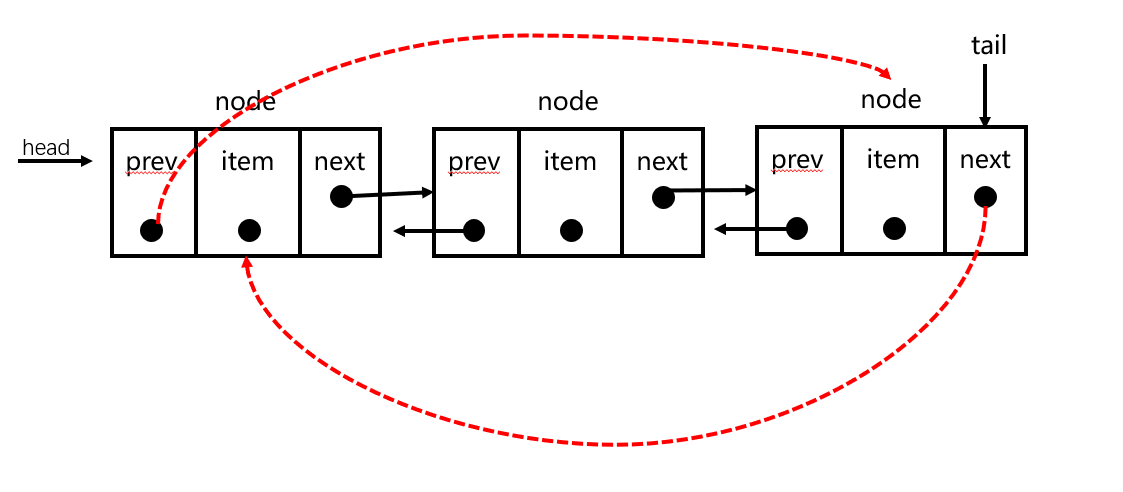## # 3. 相关题目

`leetcode`上关于链表的几道题目，附上ac代码

### # 3.1 反转链表 (opens new window)（链接直达噢！）``````var reverseList = function(head) {
let prev = null;
while (current) {
const next = current.next;
current.next = prev;
prev = current;
current = next;
}
return prev
};
``````
1
2
3
4
5
6
7
8
9
10
11

### # 3.2 合并K个升序链表 (opens new window)``````var mergeKLists = function (lists) {
if (!lists || !lists.length) return null;
let end = lists
let i = 1;
while (i != lists.length) {
end = mergeTwoLists(lists[i++], end)
}
return end
};

function mergeTwoLists(l1, l2) {
// 如果其中一个为空，直接返回另一个链表
if (!l1) return l2
if (!l2) return l1
// 依次比较链表的值
if (l1.val > l2.val) {
// 如果l2的值大，则从l2开始，l2的next通过递归再次判断是谁
l2.next = mergeTwoLists(l1, l2.next)
return l2
} else {
l1.next = mergeTwoLists(l1.next, l2)
return l1
}
};
``````
1
2
3
4
5
6
7
8
9
10
11
12
13
14
15
16
17
18
19
20
21
22
23
24

### # 3.3 删除链表的倒数第 N 个结点 (opens new window)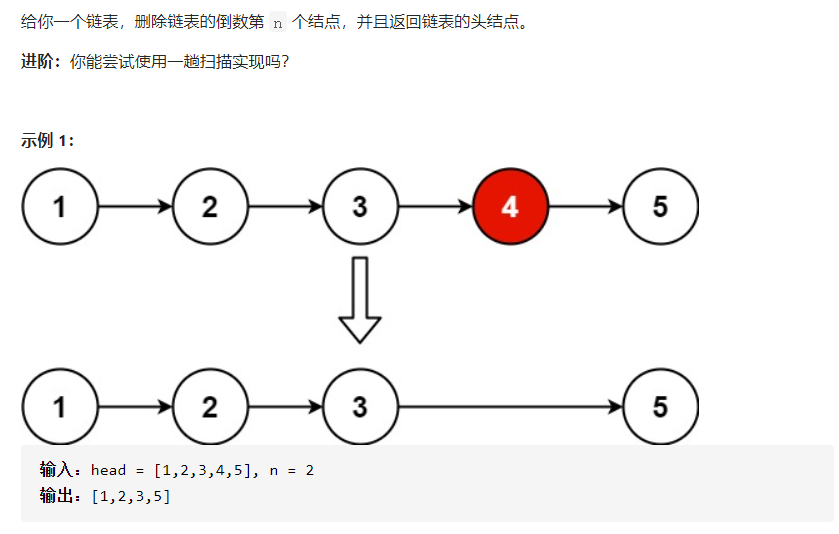``````var removeNthFromEnd = function (head, n) {
let count = 0 ;
//计算链表长度
while(node) {
node = node.next;
count++;
}
count = count - n - 1
if(count == -1) {
}else {
while(count > 0) {
node = node.next;
count--;
}
}
node.next = node.next.next
};
``````
1
2
3
4
5
6
7
8
9
10
11
12
13
14
15
16
17
18
19
20
21

## # 4. 小结

lastUpdate: 5/22/2022, 4:34:51 PM